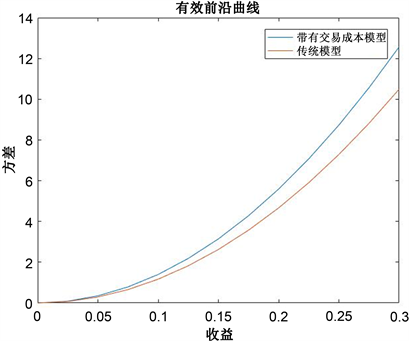﻿ 考虑交易成本的均值–方差模型的云南特色股票研究

# 考虑交易成本的均值–方差模型的云南特色股票研究A Study of Yunnan Characteristic Stocks with the Mean-Variance Model Considering Transaction Costs

Abstract: The mean-variance model is used to form a portfolio of eight representative Yunnan characteristic stocks, namely Yunnan Aluminium, the Pacific Securities, KPC Pharmaceuticals, Yunnan Nantian Electronics Information, Walvax Biotechnology, Yunnan Tourism, Yunnan Yuntianhua and 5i5j Holding Group. The daily closing price of the stock on September 9, 2019 at solstice on December 31, 2020 is used as sample data. With the help of MATLAB software, the mean value, standard deviation, variance and covariance matrix of each stock’s return rate are respectively calculated. Lagrange multiplier method is used to solve the model, and the optimal portfolio solution of the traditional Markowitz mean-variance model is obtained. Based on the investment reality, transaction costs and static capital structure factors are introduced. The traditional mean-variance model is further optimized to obtain the optimal portfolio scheme of the selected eight Yunnan characteristic stocks.

1. 引言

2. Markowitz均值–方差模型的建立

2.1. 马科维茨投资组合模型

1952年3月，Markowitz发表了《证券组合选择》的论文 ，作为现代证券组合管理理论的开端，并对风险和收益进行了量化，建立均值方差模型，提出了确定最优的资产组合基本模型。均值，是指投资组合的期望收益率，它是单只证券的期望收益率的加权平均，权重为相应的投资比例；方差是指投资组合的收益率的方差，而证券收益率的标准差称为波动率，体现了投资组合的风险。通俗来说，最优的投资组合就是给定期望风险水平下对期望收益进行最大化，或者在给定期望收益水平下对期望风险进行最小化。那在预先确定一个期望收益率，通过计算确定每种资产的权重，使其投资风险最小，所以在不同的期望收益率下得到相应的最小方差的组合解就是我们的有效组合。由此，对最优的投资组合问题求解，即是固定预期收益率为常数，权重之和为1的限制条件下求最小方差的线性规划问题。

2.2. 均值–方差模型假设

1) 风险资产的收益率符合正态分布；

2) 投资组合的风险资产之间没有很高的关联性；

3) 允许投资者卖空股票；

4) 投资者是根据证券的期望收益率估测证券组合的风险。

2.3. 均值–方差模型建立

2.3.1. 模型建立 

$\begin{array}{l}\mathrm{min}\frac{1}{2}\underset{i=1}{\overset{n}{\sum }}\underset{j=1}{\overset{n}{\sum }}{x}_{i}{x}_{j}Cov\left({r}_{i},{r}_{j}\right)\\ \text{s}\text{.t}\text{\hspace{0.17em}}\left\{\begin{array}{l}\underset{i=1}{\overset{n}{\sum }}{x}_{i}{\stackrel{¯}{r}}_{i}=\mu \\ \underset{i=1}{\overset{n}{\sum }}{x}_{i}=1\end{array}\end{array}$

$\begin{array}{l}\mathrm{min}\frac{1}{2}{x}^{\text{T}}\Sigma x\\ \text{s}\text{.t}\text{.}\text{\hspace{0.17em}}\left\{\begin{array}{l}{x}^{\text{T}}r=\mu \\ {x}^{\text{T}}{1}_{n}=1\end{array}\end{array}$

$\Sigma =\left[\begin{array}{ccc}Cov\left({r}_{1},{r}_{1}\right)& \cdots & Cov\left({r}_{1},{r}_{1}\right)\\ ⋮& \ddots & ⋮\\ Cov\left({r}_{n},{r}_{1}\right)& \cdots & Cov\left({r}_{n},{r}_{n}\right)\end{array}\right],x=\left[\begin{array}{c}{x}_{1}\\ ⋮\\ {x}_{2}\end{array}\right],r=\left[\begin{array}{c}{\stackrel{¯}{r}}_{1}\\ ⋮\\ {\stackrel{¯}{r}}_{n}\end{array}\right],{1}_{n}=\left[\begin{array}{c}1\\ ⋮\\ 1\end{array}\right]$

$\begin{array}{c}Var\left[\frac{1}{2}X+\frac{1}{2}Y\right]=\frac{1}{4}Var\left[X\right]+\frac{1}{4}Var\left[Y\right]+\frac{1}{2}Cov\left(X,Y\right)\\ \le \frac{1}{4}Var\left[X\right]+\frac{1}{4}Var\left[Y\right]+\frac{1}{2}{\sigma }_{X}{\sigma }_{Y}\\ \le \frac{1}{2}Var\left[X\right]+\frac{1}{2}Var\left[Y\right]\end{array}$

$\Delta =\left\{{x}_{i}\cdots {x}_{n}\in ℝ:{\sum }_{i=1}^{n}{x}_{i}{\stackrel{¯}{r}}_{i}=\mu ,{\sum }_{i=1}^{n}{x}_{i}=1\right\}$

3. 带有交易成本的均值–方差模型

3.1. 模型简介

3.2. 模型建立和求解

${R}_{i}=\frac{{P}_{i1}-{P}_{i0}}{{P}_{i1}}$ 为第i支股票根据市场价获得的收益率，则有：

${R}_{i}=\frac{1-\alpha }{\left(1-\beta \right)\left(1+\alpha \right)}{R}_{i}-\frac{2\alpha }{\left(1-\beta \right)\left(1+\alpha \right)}-\frac{\beta }{1-\beta }R$

${{R}^{\prime }}_{p}=\frac{1-\alpha }{\left(1-\beta \right)\left(1+\alpha \right)}{R}_{p}-\frac{2\alpha }{\left(1-\beta \right)\left(1+\alpha \right)}-\frac{\beta }{1-\beta }R$

${\left({{\sigma }^{\prime }}_{p}\right)}^{2}=\frac{{\left(1-\alpha \right)}^{2}}{{\left(1-\beta \right)}^{2}{\left(1+\alpha \right)}^{2}}{\sigma }_{p}^{2}$

$u=\frac{1-\alpha }{\left(1-\beta \right)\left(1+\alpha \right)},v=\frac{2\alpha }{\left(1-\beta \right)\left(1+\alpha \right)}+\frac{\beta }{1-\beta }R$

$\begin{array}{l}\mathrm{min}{\sigma }^{2}={u}^{2}{x}^{\text{T}}\sum x\\ \text{s}\text{.t}.\text{\hspace{0.17em}}\left\{\begin{array}{l}u{x}^{\text{T}}r-v={{R}^{\prime }}_{p}=\mu \\ {x}^{\text{T}}{1}_{n}=1\end{array}\end{array}$Figure 1. Contrast figure of effective frontier curve

4. Markowitz均值–方差模型的实证研究分析

4.1. 样本选择及数据处理Table 1. The mean, standard deviation, variance, and covariance matrix of the logarithmic returns of the eight stocks

4.2. 计算各支股票的最优投资权重

$r=\left(-0.000288\text{\hspace{0.17em}}\text{\hspace{0.17em}}0.000464\text{\hspace{0.17em}}\text{\hspace{0.17em}}-0.000428\text{\hspace{0.17em}}\text{\hspace{0.17em}}-0.000165\text{\hspace{0.17em}}\text{\hspace{0.17em}}0.000408\text{\hspace{0.17em}}\text{\hspace{0.17em}}0.000126\text{\hspace{0.17em}}\text{\hspace{0.17em}}0.000108\text{\hspace{0.17em}}\text{\hspace{0.17em}}-0.000203\right)$

${1}_{n}={\left(1,1,1,1,1,1,1,1\right)}^{\prime }$

$\sum =\left(\begin{array}{l}0.000141\text{\hspace{0.17em}}\text{\hspace{0.17em}}\text{ }0.000049\text{\hspace{0.17em}}\text{\hspace{0.17em}}0.000043\text{\hspace{0.17em}}\text{\hspace{0.17em}}0.000056\text{\hspace{0.17em}}\text{\hspace{0.17em}}0.000023\text{\hspace{0.17em}}\text{\hspace{0.17em}}0.000042\text{\hspace{0.17em}}\text{\hspace{0.17em}}0.000038\text{\hspace{0.17em}}\text{\hspace{0.17em}}0.000044\hfill \\ 0.000049\text{\hspace{0.17em}}\text{\hspace{0.17em}}0.000174\text{\hspace{0.17em}}\text{\hspace{0.17em}}0.000044\text{\hspace{0.17em}}\text{\hspace{0.17em}}0.000054\text{\hspace{0.17em}}\text{\hspace{0.17em}}0.000026\text{\hspace{0.17em}}\text{\hspace{0.17em}}0.000074\text{\hspace{0.17em}}\text{\hspace{0.17em}}0.000058\text{\hspace{0.17em}}\text{\hspace{0.17em}}0.000051\hfill \\ 0.000043\text{\hspace{0.17em}}\text{\hspace{0.17em}}0.000044\text{\hspace{0.17em}}\text{\hspace{0.17em}}0.000081\text{\hspace{0.17em}}\text{\hspace{0.17em}}0.000046\text{\hspace{0.17em}}\text{\hspace{0.17em}}0.000052\text{\hspace{0.17em}}\text{\hspace{0.17em}}0.000037\text{\hspace{0.17em}}\text{\hspace{0.17em}}0.000030\text{\hspace{0.17em}}\text{\hspace{0.17em}}0.000020\hfill \\ 0.000056\text{\hspace{0.17em}}\text{\hspace{0.17em}}0.000054\text{\hspace{0.17em}}\text{\hspace{0.17em}}0.000046\text{\hspace{0.17em}}\text{\hspace{0.17em}}0.000140\text{\hspace{0.17em}}\text{\hspace{0.17em}}0.000037\text{\hspace{0.17em}}\text{\hspace{0.17em}}0.000067\text{\hspace{0.17em}}\text{\hspace{0.17em}}0.000048\text{\hspace{0.17em}}\text{\hspace{0.17em}}0.000043\hfill \\ 0.000023\text{\hspace{0.17em}}\text{\hspace{0.17em}}0.000026\text{\hspace{0.17em}}\text{\hspace{0.17em}}0.000052\text{\hspace{0.17em}}\text{\hspace{0.17em}}0.000037\text{\hspace{0.17em}}\text{\hspace{0.17em}}0.000224\text{\hspace{0.17em}}\text{\hspace{0.17em}}0.000031\text{\hspace{0.17em}}\text{\hspace{0.17em}}0.000034\text{\hspace{0.17em}}\text{\hspace{0.17em}}0.000015\hfill \\ 0.000042\text{\hspace{0.17em}}\text{\hspace{0.17em}}0.000074\text{\hspace{0.17em}}\text{\hspace{0.17em}}0.000037\text{\hspace{0.17em}}\text{\hspace{0.17em}}0.000067\text{\hspace{0.17em}}\text{\hspace{0.17em}}0.000031\text{\hspace{0.17em}}\text{\hspace{0.17em}}0.000132\text{\hspace{0.17em}}\text{\hspace{0.17em}}0.000043\text{\hspace{0.17em}}\text{\hspace{0.17em}}0.000032\hfill \\ 0.000038\text{\hspace{0.17em}}\text{\hspace{0.17em}}0.000058\text{\hspace{0.17em}}\text{\hspace{0.17em}}0.000030\text{\hspace{0.17em}}\text{\hspace{0.17em}}0.000048\text{\hspace{0.17em}}\text{\hspace{0.17em}}0.000034\text{\hspace{0.17em}}\text{\hspace{0.17em}}0.000043\text{\hspace{0.17em}}\text{\hspace{0.17em}}0.000104\text{\hspace{0.17em}}\text{\hspace{0.17em}}0.000035\hfill \\ 0.000044\text{\hspace{0.17em}}\text{\hspace{0.17em}}0.000051\text{\hspace{0.17em}}\text{\hspace{0.17em}}0.000020\text{\hspace{0.17em}}\text{\hspace{0.17em}}0.000043\text{\hspace{0.17em}}\text{\hspace{0.17em}}0.000015\text{\hspace{0.17em}}\text{\hspace{0.17em}}0.000032\text{\hspace{0.17em}}\text{\hspace{0.17em}}0.000035\text{\hspace{0.17em}}\text{\hspace{0.17em}}0.000113\hfill \end{array}\right)$

$x={\left[-12.0806\text{\hspace{0.17em}}\text{\hspace{0.17em}}88.2476\text{\hspace{0.17em}}\text{\hspace{0.17em}}-149.6723\text{\hspace{0.17em}}\text{\hspace{0.17em}}-7.3367\text{\hspace{0.17em}}\text{\hspace{0.17em}}66.6058\text{\hspace{0.17em}}\text{\hspace{0.17em}}21.1144\text{\hspace{0.17em}}\text{\hspace{0.17em}}29.8224\text{\hspace{0.17em}}\text{\hspace{0.17em}}-35.7006\right]}^{\prime }$

${x}^{*}=\frac{x}{‖x‖}$

${x}^{*}={\left[-0.0294\text{\hspace{0.17em}}\text{\hspace{0.17em}}0.2149\text{\hspace{0.17em}}\text{\hspace{0.17em}}-0.3645\text{\hspace{0.17em}}\text{\hspace{0.17em}}-0.0179\text{\hspace{0.17em}}\text{\hspace{0.17em}}0.1622\text{\hspace{0.17em}}\text{\hspace{0.17em}}0.0514\text{\hspace{0.17em}}\text{\hspace{0.17em}}0.0726\text{\hspace{0.17em}}\text{\hspace{0.17em}}-0.0870\right]}^{\prime }$

(负数表示该股票可以卖空)Table 2. Optimal investment weight derived from the model

5. 带有资本结构因子和交易成本的Markowitz均值–方差模型的实证研究分析

5.1. 由上一节求得的样本数据进行运算

$x={\left[-12.6656\text{\hspace{0.17em}}\text{\hspace{0.17em}}92.4976\text{\hspace{0.17em}}\text{\hspace{0.17em}}-156.8940\text{\hspace{0.17em}}\text{\hspace{0.17em}}-7.6895\text{\hspace{0.17em}}\text{\hspace{0.17em}}69.8092\text{\hspace{0.17em}}\text{\hspace{0.17em}}22.1248\text{\hspace{0.17em}}\text{\hspace{0.17em}}31.2486\text{\hspace{0.17em}}\text{\hspace{0.17em}}-37.4311\right]}^{\prime }$

${x}^{*}=\frac{x}{‖x‖}$

${x}^{*}={\left[-0.0294\text{\hspace{0.17em}}\text{\hspace{0.17em}}0.2149\text{\hspace{0.17em}}\text{\hspace{0.17em}}-0.3646\text{\hspace{0.17em}}\text{\hspace{0.17em}}-0.0179\text{\hspace{0.17em}}\text{\hspace{0.17em}}0.1622\text{\hspace{0.17em}}\text{\hspace{0.17em}}0.0514\text{\hspace{0.17em}}\text{\hspace{0.17em}}0.0726\text{\hspace{0.17em}}\text{\hspace{0.17em}}-0.0870\right]}^{\prime }$

(负数表示该股票可以卖空)Table 3. Optimal Investment Weight derived from the model

5.2. 计算在不同预期收益率下的最优投资组合

${\mu }_{1}=0.05,{\mu }_{2}=0.075,{\mu }_{3}=0.10,{\mu }_{4}=0.125,{\mu }_{5}=0.15,{\mu }_{6}=0.175,{\mu }_{7}=0.20$ 分别计算其相应的最优投资组合，相应结果如表4所示：Table 4. Optimal portfolio under different expected returns

6. 结束语

NOTES

*通讯作者。

 Markowitz, H.M. (1952) Portfolio Selection. The Journal of Finance, 7, 77-91.
https://doi.org/10.1111/j.1540-6261.1952.tb01525.x

 李雄英, 王斌会. 稳健均值-方差模型的构建及比较研究[J]. 统计与决策, 2020, 36(13): 47-52.

 王晓琴, 高岳林. 带有交易成本的均值-方差-下半方差投资组合模型[J]. 工程数学学报, 2020, 37(2): 155-164.

 王晓琴, 高岳林. 考虑交易费用的均值-VaR多阶段投资组合优化模型[J]. 工程数学学报, 2020, 37(6): 673-684.

 刘勇军, 周敏娜, 张卫国. 考虑背景风险的均值-半方差投资组合优化模型[J]. 系统工程理论与实践, 2020, 40(9): 2282-2291.

 黄德春, 张长征, 汤云超. 含资本结构因子和交易费用的CVaR投资组合模型分析[J]. 经济与管理, 2009, 23(9): 33-37.

 吴萌, 黄南京, 赵昌文. 一个扩展的含资本结构因子和交易成本的证券组合投资模型[J]. 四川大学学报(自然科学版), 2005, 42(4): 639-643.

 李宏杰. 具有资本结构因子和交易成本的证券组合投资模型[J]. 中国管理科学, 2007, 15(3): 14-18.

Top